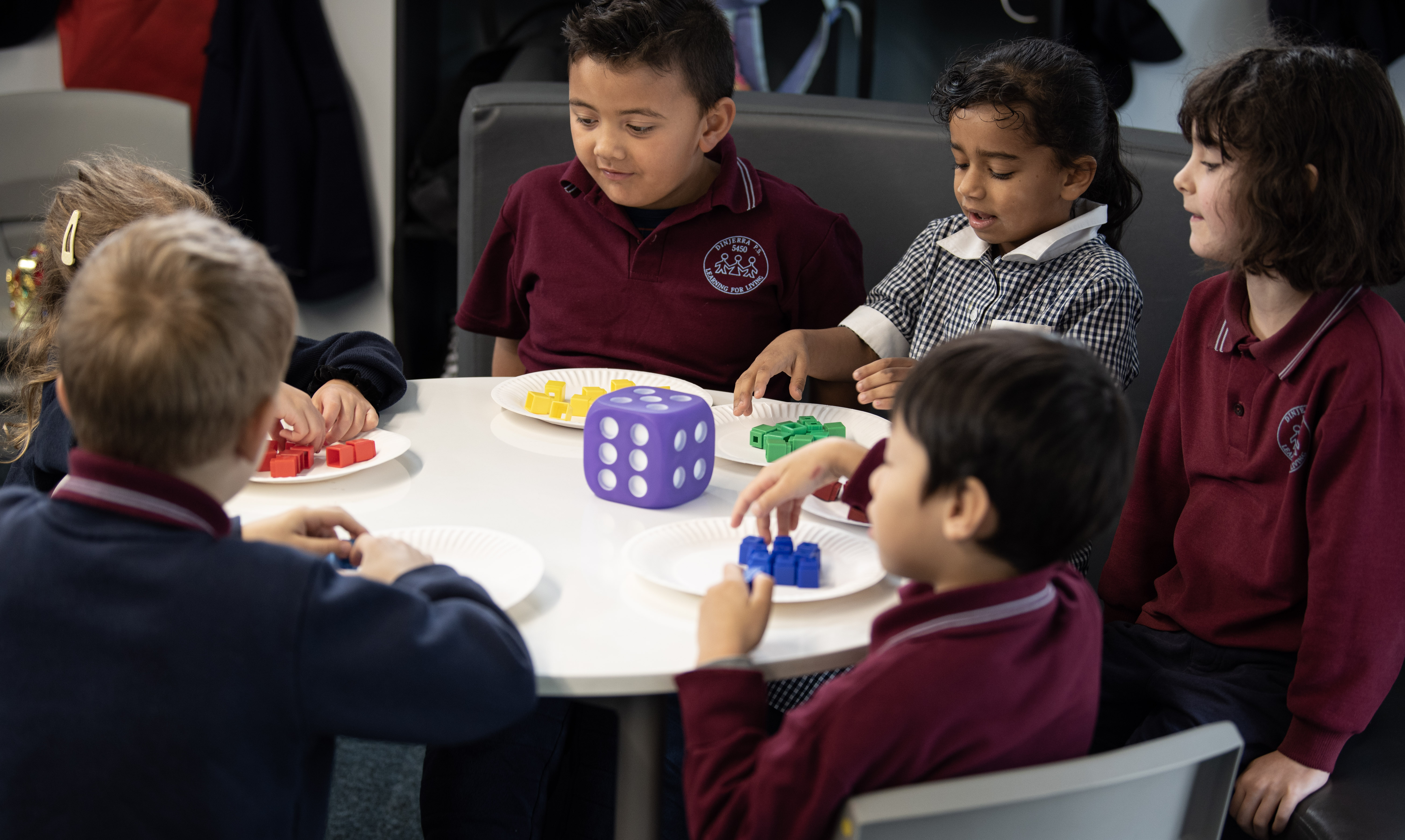# MathematicsAt DinjerraPrimary School we see mathematics as an integral part of life learning. We encourage students to see maths as fun and as an opportunity to challenge themselvesand succeed. We promote mathematics in a positive way and work to ensure all students have a sound grasp of mathematic strategies and understandings.

Within the Australian Curriculum mathematics is organised around the interaction of three content strandsand four proficiency strands.

### Content

The content strands are Number and Algebra, Measurement and Geometry, and Statistics and Probability.They describe what is to be taught and learnt.

### Proficiency

The proficiency strands are Understanding, Fluency, Problem Solving, and Reasoning.They describe how content is explored or developed, that is, the thinking and doing of mathematics.This approach has been adopted to ensure students’ proficiency in mathematical skills develops throughout the curriculum and becomes increasingly sophisticated over the years of schooling.

### Content Strands

Number and Algebra

Number and Algebra are developed together, as each enriches the study of the other. Students apply number sense and strategies for counting and representing numbers. They explore the magnitude and properties of numbers. They apply a range of strategies for computation and understand the connections between operations. They build on their understanding of the number system to describe relationships and formulate generalisations. They recognise equivalence and solve equations and inequalities. They apply their number and algebra skills to conduct investigations, solve problems and communicate their reasoning.

Measurement and Geometry

Measurement and Geometry are presented together to emphasise their relationship to each other, enhancing their practical relevance. Students develop an increasingly sophisticated understanding of size, shape, relative position and movement of two-dimensional figures and three-dimensionalobjects. They investigate properties and apply their understanding to define, compare and construct figures and objects. They learn to develop geometric arguments. They make meaningful measurements of quantities, choosing appropriate metric units of measurement.

Statistics and Probability

Statistics and Probability initially develop in parallel and the curriculum then progressively builds the links between them. Students recognise and analyse data and draw inferences. They represent, summarise and interpret data and undertake purposeful investigations involving the collection and interpretation of data. They assess likelihood and assign probabilities using experimental and theoretical approaches.

Proficiency Strands

The proficiency strands describe the actions in which students can engage when learning and using the content. While not all proficiency strands apply to every content description, they indicate the breadth of mathematical actions that teachers can emphasise. The proficiency strands are; Understanding, Fluency, Problem Solving, and Reasoning.